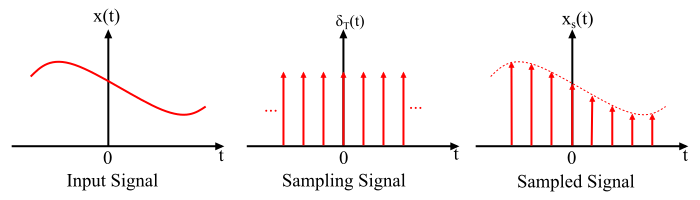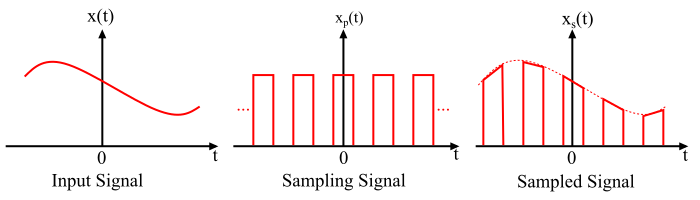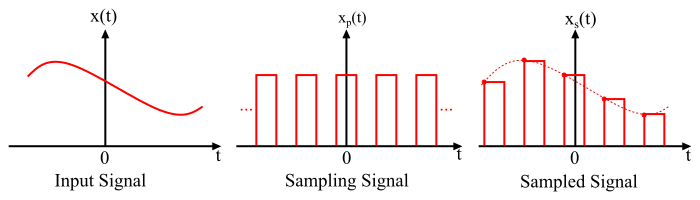# Different Types of Sampling Techniques

## Sampling

The process of converting a continuous-time signal into a discrete-time signal is called the sampling.

After sampling, the signal is defined at discrete-instants of time and the time interval between two successive sampling instants is called the sampling period.

## Sampling Techniques

The sampling of a signal is done in several ways. Generally, there are three types of sampling techniques viz. −

• Instantaneous Sampling or Impulse Sampling
• Natural Sampling
• Flat Top Sampling

Here, the instantaneous sampling or impulse sampling is also called the ideal sampling, whereas the natural sampling and flat top sampling are called the practical sampling techniques. These three sampling methods are explained as follows −

## Ideal Sampling

• Ideal sampling is also known as instantaneous or impulse train sampling.
• In this method, the sampling signal is a periodic impulse train.
• The area of each impulse in the sampled signal is equal to the instantaneous value of the input signal x(t).

### Ideal Sampling – Sampler Circuit### Ideal Sampling – Process of Sampling## Natural Sampling

• Natural sampling is also called practical sampling.
• In this sampling technique, the sampling signal is a pulse train.
• In natural sampling method, the top of each pulse in the sampled signal retains the shape of the input signal x(t) during pulse interval.

### Natural Sampling – Sampler Circuit### Natural Sampling – Process of Sampling## Flat Top Sampling

• The flat top sampling is also the practical sampling technique.
• In the flat top sampling, the sampling signal is also a pulse train.
• The top of each pulse in the sampled signal remain constant and is equal to the instantaneous value of the input signal 𝑥(𝑛) at the start of the samples.

### Flat Top Sampling – Sampler Circuit### Flat Top Sampling – Sampling Process